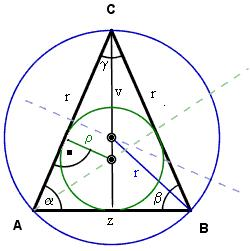# Calculate 3292

The perimeter of the isosceles triangle is 32 cm. The base is 2 cm longer than the shoulder. Calculate the sides of the triangle.

a =  10
b =  10
c =  12

### Step-by-step explanation:

a=b
c = 2+a
a+b+c = 32

a-b = 0
a-c = -2
a+b+c = 32

Row 2 - Row 1 → Row 2
a-b = 0
b-c = -2
a+b+c = 32

Row 3 - Row 1 → Row 3
a-b = 0
b-c = -2
2b+c = 32

Pivot: Row 2 ↔ Row 3
a-b = 0
2b+c = 32
b-c = -2

Row 3 - 1/2 · Row 2 → Row 3
a-b = 0
2b+c = 32
-1.5c = -18

c = -18/-1.5 = 12
b = 32-c/2 = 32-12/2 = 10
a = 0+b = 0+10 = 10

a = 10
b = 10
c = 12

Our linear equations calculator calculates it.Did you find an error or inaccuracy? Feel free to write us. Thank you!

Tips for related online calculators
Do you have a linear equation or system of equations and looking for its solution? Or do you have a quadratic equation?
Calculation of an isosceles triangle.